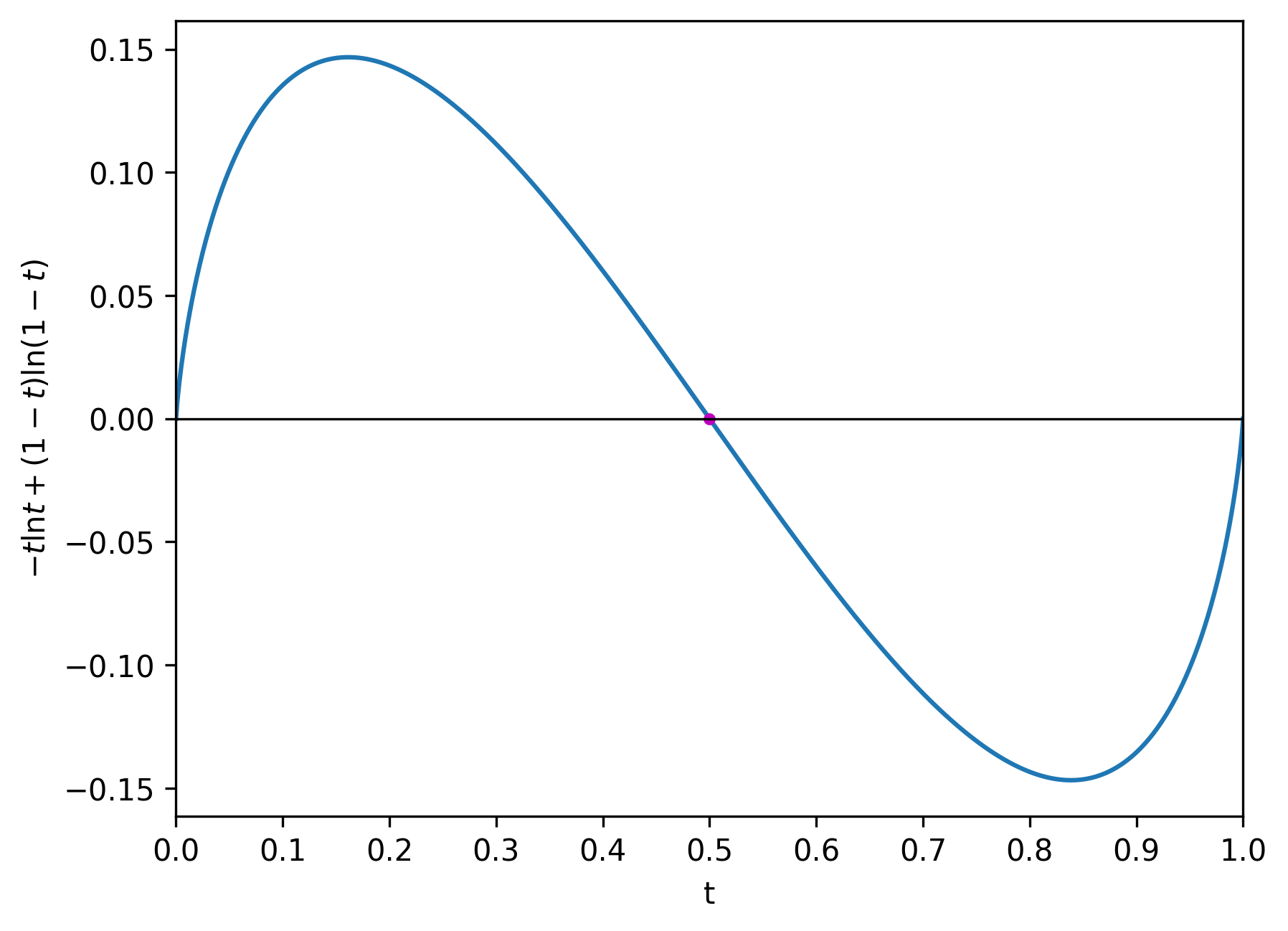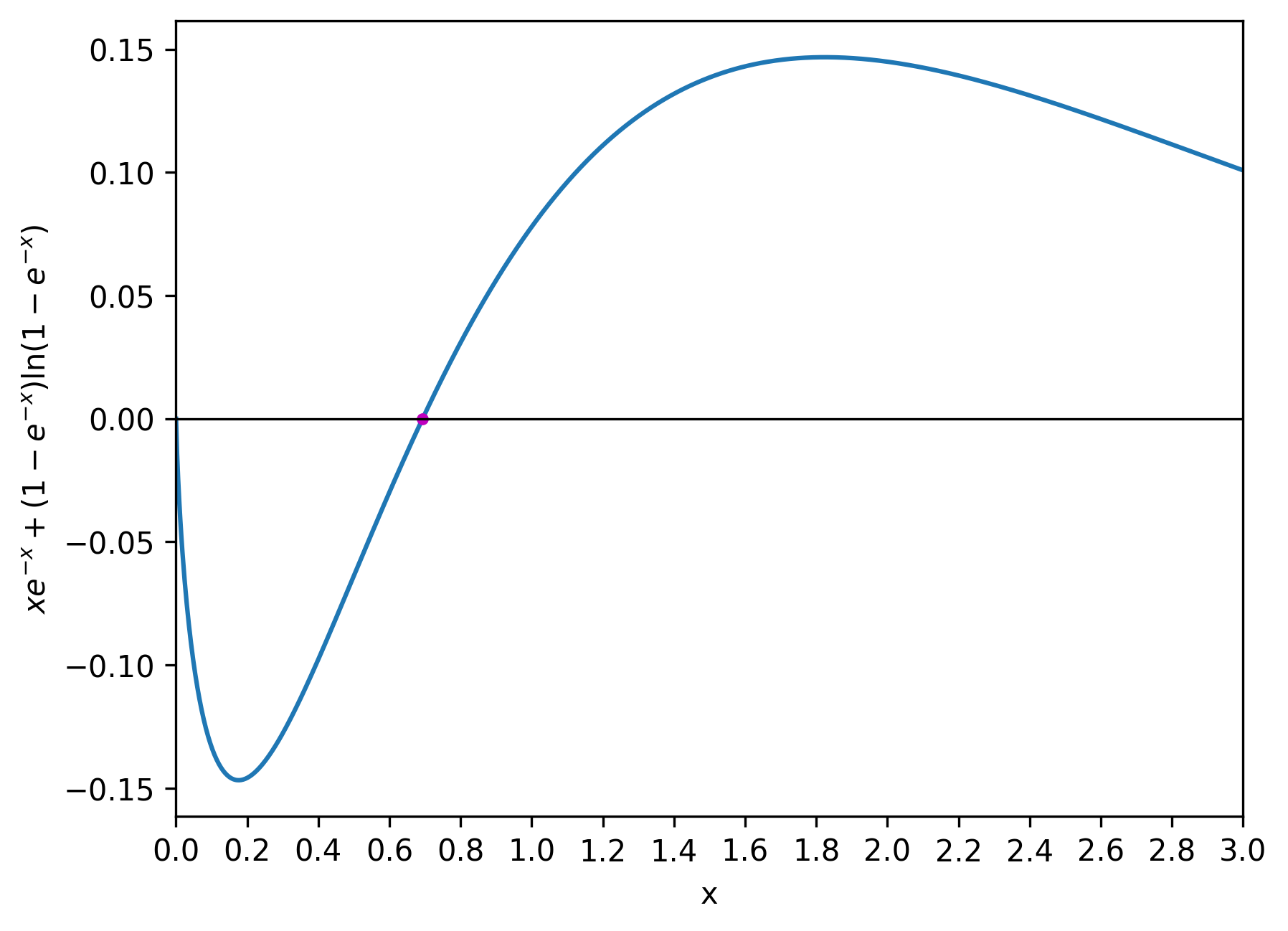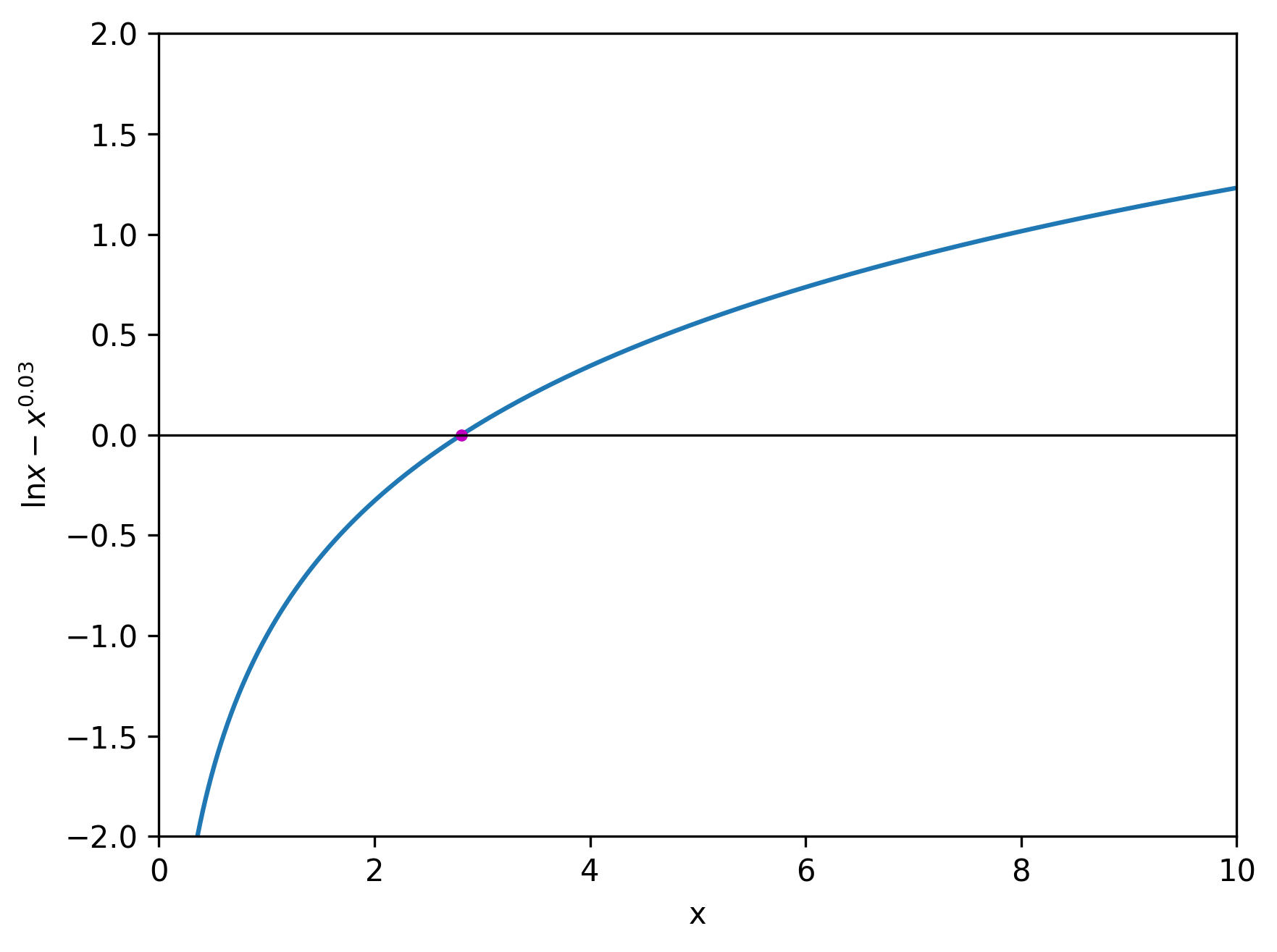# Clumsy Equations

By Susam Pal on 28 Oct 2010

## Less Clumsy Equation

At work today, while calculating optimal parameters for a data structure, I arrived at this equation: $x e^{-x} + (1 - e^{-x}) \ln (1 - e^{-x}) = 0.$ This equation is a little clumsy to deal with. Let us make this neat by substituting $$e^{-x}$$ with $$t.$$ Now we get $-t \ln t + (1 - t) \ln (1 - t) = 0.$ If we rearrange this equation as $t \ln t = (1 - t) \ln (1 - t),$ it is easy to see that one of the solutions is $$t = 1 - t,$$ thus $$t = \frac{1}{2}.$$ Are there more solutions to this equation? The answer turns out to be: no.Graph of the function $$f(t) = -t \ln t + (1 - t) \ln (1 - t)$$

In this problem, $$x$$ is a real number and so is $$t.$$ In the domain of real numbers, $$\ln t$$ is undefined for $$t \le 0.$$ As a result, $$\ln (1 - t)$$ is undefined for $$t \ge 1.$$ Therefore we need to look for values of $$t$$ in the open interval $$(0, 1)$$ that could be solutions to the equation.

The function $$\ln t$$ is a strictly increasing function for $$t > 0.$$ This is easy to show from the derivative of $$\ln t.$$ The derivative of $$\ln t$$ is $$\frac{1}{t}$$ which is strictly positive for $$t > 0.$$

Since $$\ln t$$ is a strictly increasing function for $$t > 0,$$ we can make the following conclusions:

• For $$0 < t < \frac{1}{2},$$ $$t < (1 - t) \implies t \ln t < (1 - t) \ln (1 - t).$$
• For $$\frac{1}{2} < t < 1,$$ $$t > (1 - t) \implies t \ln t > (1 - t) \ln (1 - t).$$

This proves that there are no values of $$t$$ in the open intervals $$\left( 0, \frac{1}{2} \right)$$ and $$\left( \frac{1}{2}, 1 \right)$$ that could be solutions to the equation. Therefore $$t = \frac{1}{2}$$ is the only solution to the equation.

Since $$t = e^{-x},$$ we now arrive at the solution to the original equation expressed in terms of $$x$$ as follows: \begin{align*} t = \frac{1}{2} & \iff e^{-x} = \frac{1}{2} \\ & \iff -x = \ln \frac{1}{2} \\ & \iff x = \ln 2 \approx 0.6931. \end{align*}Graph of the function $$f(x) = x e^{-x} + (1 - e^{-x}) \ln (1 - e^{-x})$$

## More Clumsy Equation

Here is another equation that I had to solve a couple of years ago: $\ln x - x^{0.03} = 0.$ There is no simple way to solve this equation. I had to resort to using the Lambert W function to solve this. To use this function, we first need to bring our equation to the form $$f(x) e^{f(x)} = Y$$ where $$f(x)$$ is a function of $$x$$ and $$Y$$ is an expression that does not contain $$x.$$ The solution of $$f(x) e^{f(x)} = Y$$ is $$f(x) = W(Y).$$ To summarize: $f(x) e^{f(x)} = Y \iff f(x) = W(Y).$ Applying this technique to our equation, we get \begin{align*} \ln x - x^{0.03} = 0 & \iff \ln x = x^{0.03} \\ & \iff \ln x = e^{0.03 \ln x} \\ & \iff (\ln x)(e^{-0.03 \ln x}) = 1 \\ & \iff (-0.03 \ln x)(e^{-0.03 \ln x}) = -0.03. \\ \end{align*} We have brought the equation to the desired $$f(x) e^{f(x)} = Y$$ form where $$f(x) = -0.03 \ln x$$ and $$Y = -0.03.$$ Therefore the solution to this equation is $-0.03 \ln x = W(-0.03).$ Simplifying this further, we get $x = e^{\frac{W(-0.03)}{-0.03}}.$ The Maclaurin series of $$W(x)$$ is given by $W(x) = \sum_{n=1}^\infty \frac{(-n)^{n-1}}{n!} x^n = x - x^2 + \frac{3}{2} x^3 - \frac{8}{3} x^4 + \frac{125}{24} x^5 - \dots$ Using the Maclaurin series of $$W(x),$$ we get $W(-0.03) \approx -0.0309428.$ Therefore $x = e^{\frac{W(-0.03)}{-0.03}} \approx e^{\frac{-0.0309428}{-0.03}} \approx 2.80506.$Graph of the function $$f(x) = \ln x - x^{0.03}$$